Home > GC > Chapter 6 > Lesson 6.2.3 > Problem6-62

6-62.
1. For each diagram below, use geometric relationships to solve for the given variable(s). Check your answer. Homework Help ✎

1.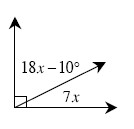2.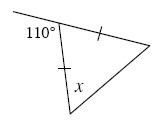3.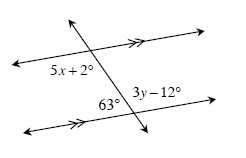4.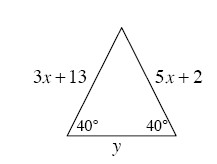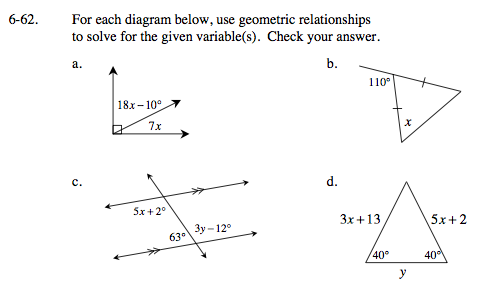The angle that is 18x - 10° and the angle 7x are complementary.
Complementary means the angles add up to _____

Adjacent angle pairs that form a straight line are supplementary and base angles of an isosceles triangle are equal.

x = 55°

First solve for y, then solve for x.

3y − 12° + 63° = 180°

Since the triangle is isosceles, start by solving for x.
Next, solve for the unknown interior angle.
Then use the Law of Sines to solve for y.

Refer to the Math Notes box in Lesson 5.3.2 if you need help remembering the Law of Sines.

$\textit{x} = \frac{11}{2}$

$\textit{y} = \frac{29.5\sin (100^{\circ})}{\sin (40^{\circ})}\approx 45.20$